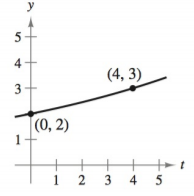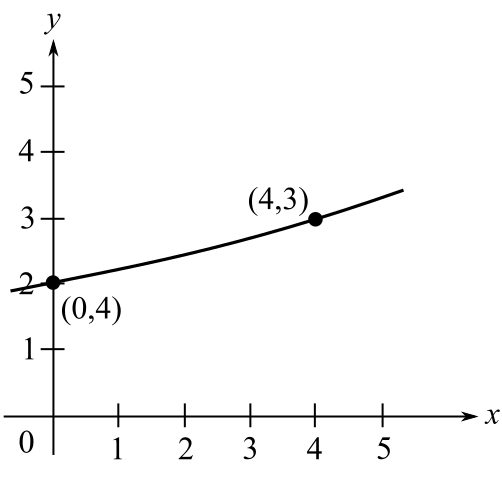Chapter 4.6, Problem 1E### Calculus: An Applied Approach (Min...

10th Edition
Ron Larson
ISBN: 9781305860919

#### Solutions

Chapter
Section### Calculus: An Applied Approach (Min...

10th Edition
Ron Larson
ISBN: 9781305860919
Textbook Problem
1 views

# Modeling Exponential Growth and Decay In Exercises 1-6, find the exponential function y = C e k t that passes through the two given points. y = C e k tTo determine

To calculate: The exponential function y=Cekt for the following graph:Explanation

Given Information:

The provided graph is:

Calculation:

Consider the following graph:

Observe that the exponential function curve passes through the points (0,2) and (4,3).

Hence the points (0,2) and (4,3) satisfy the equation of exponential function y=Cekt.

Substitute t=0,y=2 in the equation y=Cekt.

2=Cek(0)2=Ce02=C1C=2

Hence, the value of C is 2.

Substitute t=4,y=3 in the equation y=2ekt.

3=2ek(4)3=2e4k

Solve the equation 2e3k=4 for k

### Still sussing out bartleby?

Check out a sample textbook solution.

See a sample solution

#### The Solution to Your Study Problems

Bartleby provides explanations to thousands of textbook problems written by our experts, many with advanced degrees!

Get Started

#### Find more solutions based on key concepts